# Probability

The CAT quantitative aptitude section includes several questions from the topic of probability. The probability for CAT questions test the mathematical as well as reasoning skills of the candidates. CAT aspirants can visit the questions page (link at the bottom) to practice various related questions and prepare more effectively for the CAT exam.

Probability can be defined as the measure of how likely an event will occur and it ranges from 0 to 1. An event is impossible if the probability is 0; while a probability of 1 indicates the event is certain to occur.

### Introduction To Probability

Probability is the likelihood or chance that an event will occur. If there is no chance that an event will occur, then its probability of occurring will be 0. On the other extreme, if an event is certain to occur, then its probability of occurring will be 100%, or 1. Hence, the probability should be a number between 0 and 1, inclusive.

Suppose your favorite actor has a 1 in 3 chance of winning the Oscar for best actor. This can be measured by forming the fraction 1/3. Hence, a probability is a fraction where the top is the number of ways an event can occur and the bottom is the total number of possible events:

Probability is represented as a pure number p in the range 0 = p = 1

Let’s take a typical example to understand the concept of probability.

Suppose you have a fair coin. It has two sides. One is a head and the other is a tail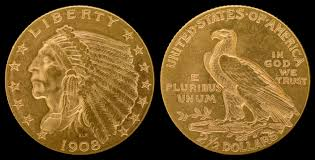Based on this, the probability of getting a result on tossing a coin can be estimated i.e., when you toss a coin the probability of getting heads or the probability of getting tails is same i.e. 1/2.

#### Explanation:

In the above example; the probability of getting neither heads nor tails on tossing a fair coin is zero; as either heads or tails has to occur.

Similarly, the probability of landing on either a head or a tail on tossing a coin is one or unity; as these two are the only possible outcomes.

Let’s now look at some more varied examples related to probability

Example: A spinner has 4 equal sectors colored yellow, blue, green and red. After spinning the spinner, what is the probability of landing on each color?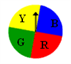Outcomes: The possible outcomes of this experiment are yellow, blue, green, and red. Probabilities of each event can be calculated as follows:

P(yellow) = (number of ways to land on yellow)/(total number of colors) = 1/4

P(blue) = (number of ways to land on blue)/(total number of colors) = 1/4

P (green) = (number of ways to land on green)/(total number of colors) = 1/4

P (red) = (number of ways to land on red)/(total number of colors) = 1/4

Thus, one can arrive at a general formula for the occurrence of an event (A) as

P(A) = (The Number Of Ways Event A Can Occur)/(The Total Number Of Possible Outcomes)

#### Probability Formula:

Probability = (Number of ways an event can occur)/(Number of total possible events)

#### Basic Probability Definitions:

 Definition Examples An experiment is a situation involving chance or probability that leads to results called outcomes. In the problem above, the experiment is tossing the coin. An outcome is the result of a single trial of an experiment. The possible outcomes are heads or tails. An event is one or more outcomes of an experiment. One event of the above experiment is landing on heads. Probability is the measure of how likely an event is. The probability of landing on tails is ½.

Here is another example-

Example: A single 6-sided die is rolled.

(a) What is the probability of each outcome?

(b) What is the probability of rolling an even number?

(c) What is the probability of rolling an odd number?

Suppose you need to calculate the probability of getting 1 on throwing a die.

Let us first look at the possible outcomes of this throw.

The possible outcomes of this experiment are 1, 2, 3, 4, 5 and 6, and all outcomes are equally likely i.e. the probability of getting a “1” is the same as any other number on the die.

Since there are 6 equally likely outcomes in a single throw of a die, there is only one way of getting a particular outcome.Therefore the probability of getting 1 on the die is 1 out of the 6 possible outcomes.

In other words, the probability of getting “1” in a single throw of a die is 1/6 Thus, you can calculate all outcomes as follows

P (even) = (Number of ways to roll an even number)/(total number of sides) = 3/6 = 1/2

P (odd) = (Number of ways to roll an odd number)/(total number of sides) = 3/6 = 1/2

P (1) = (number of ways to roll at 1)/(total number of sides) = 1/6

P (2) = (number of ways to roll at 2)/(total number of sides) = 1/6

P (3) = (number of ways to roll at 3)/(total number of sides) = 1/6 …and so on.

### Definitions Related to Probability

• Sample Space in Probability:

A sample space in probability is the set of all possible outcomes of a particular experiment. Consider the experiment of flipping two coins The sample space will be {HH, HT, TH, TT }

where H ⇒ Head and T ⇒ Tail

An event is a subset of the sample space.

For eg) the event that a head shows up will be {HH, HT, TH}

Also, The sum of all the probabilities in the sample space is always 1.

• Complement of an Event:

If E is an event within the sample space S of an activity or experiment, the complement of E (denoted E’) consists of all outcomes in S that are not in E. The complement of E is everything else in the problem that is NOT in E.

Example:

Experiment: Tossing a coin

Event E ⇔ The coin shows heads.

Complement (E’) ⇒ The coin shows tails.

The probability of the complement of an event is one minus the probability of the event.

∴ P(E’) = 1 – P(E)

### Question: A pair of dice is rolled. What is the probability of not rolling doubles?

Solution:

Sample space= rolling of two dies = 6 × 6= 36 Doubles are (1, 1), (2, 2), (3, 3), (4, 4), (5, 5), (6, 6)

P(doubles) = 6/36 = 1/6 P(not doubles).

So, according to the law of probability, P(not doubles) = 1 – 1/6 = 5/6.

Question: A pair of dice is rolled. What is the probability of rolling 9 or less?

Solution:

Sample space= rolling of two dies = 6 × 6 = 36.

The complement of rolling “9 or less” is rolling 10, 11 or 12.

∴ P(9 or less) = 1 – P(10, 11 or 12) = 1 – [P(10) + P(11) + P(12)] (to get a 10 there are 3 ways (6, 4),(4, 6) and (5, 5) and to get a 11, there are 2 ways (6,5) and (5, 6) & to get a 12, there is only one way (6, 6)) = 1 – (3/36 + 2/36 + 1/36) = 5/36.

• Mutually exclusive events

Two events are mutually exclusive if they cannot occur at the same time (i.e., they have no outcomes in common).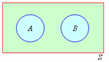Example:

What’s the probability of getting either heads or tails when flipping a coin once?

Since the only possible outcomes are heads or tails, we expect the probability to be 100%, or 1/2 : 1/2 = 1.

The events Heads and tails are mutually exclusive. That is, if heads occurs, then tails cannot (and vice versa).

For any two mutually exclusive events, the probability that an outcome will be in one event or the other event is the sum of their individual probabilities. Thus, a new law of probabilitty can be derived as below.

If A and B are mutually exclusive events,

=> P(A or B) = P(A U B) = P(A) + P(B)

#### Examples of Mutually Exclusive Events:

Question: A pair of dice is rolled. What is the probability that the sum of the numbers rolled is either 7 or 11?

Solution:

Sample space= rolling of two dies = 6 × 6= 36 Six outcomes have a sum of 7:(1, 6), (2, 5), (3, 4), (4, 3), (5, 2), (6, 1) P(7) = 6/36

Two outcomes have a sum of 11: (5, 6), (6, 5) P(11) = 2/36 As you can observe, the sum of the numbers cannot be 7 and 11 at the same time, so these events are mutually exclusive. P (7 or 11) = P(7) + P(11) = 6/36 + 2/36 = 8/36 = 2/9

When 2 events are not mutually exclusive, there will be some overlapping between events as shown below. We now need to consider the overlapped region as well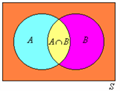For any two events, which are not mutually exclusive, the probability that an outcome will be in one event or the other event is the sum of their individual probabilities minus the probability of the outcome being in both events.

If events A and B are NOT mutually exclusive,

P(A or B) = P(A U B) = P(A) + P(B) – P(A ∩ B)

Where A ∩ B is the occurrence of A and B.

Question: In the throw of a die, find the probability of getting an even number or a prime number

Solution:

The probability of getting an even number (2, 4, 6) = 3/6 = 1/2. The probability of getting a prime number (2, 3, 5)= 3/6 = 1/2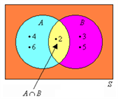Probability of getting a number which is both even and prime (2) = 1/6 P(even or prime) = P(even) + P(prime) – P(even and prime) = 1/2 + 1/2 – 1/6 = 5/6.

• Independent Events

Two events are said to be independent if the result of the second event is not affected by the result of the first event. If A and B are independent events, the probability of both events occurring is the product of the probabilities of the individual events. From this, a different law of probability can be deduced i.e.,

∴ If A and B are independent events,

⇒ P(A and B) = P(A) × P(B).

#### Example of Independent Events:

A drawer contains 3 red balls, 4 green balls, and 5 blue balls. One ball is taken from the drawer and then replaced. Another ball is taken from the drawer. What is the probability that the first ball is red and the second ball is blue?

Because the first ball is replaced, the sample space of 12 balls does not change from the first event to the second event. The events are independent. P (red followed by blue) = P (red) × P(blue) = 3/12 × 5/12 = 15/144 = 5/48.

• Dependent Events

If the result of one event is affected by the result of another event, the events are said to be dependent. If A and B are dependent events, the probability of both events occurring is the product of the probability of the first event and the probability of the second event once the first event has occurred. If A and B are dependent events, and A occurs first,

∴P(A and B) = P(A)× P(B, once A has occurred)

#### Example of Dependent Events:

A drawer contains 3 red balls, 4 green balls, and 5 blue balls. One ball is taken from the drawer and is NOT replaced. Another ball is taken from the drawer. What is the probability that the first ball is red and the second ball is blue?

Because the first ball is NOT replaced, the sample space of the second event is changed. The sample space of the first event is 12 balls, but the sample space of the second event is now 11 balls. The events are thus dependent. P (red then blue) = P (red) × P (blue) = 3/12×5/11 = 15/132=5/44.

### Practice Probability Questions:

1) A pair of dice is rolled. Two possible events are rolling a number greater than 8 and rolling an even number. Are these two events mutually exclusive events?

2) A pair of dice is rolled. A possible event is rolling a multiple of 5. What is the probability of the complement of this event?

3) A paper bag contains 15 cubes of equal size. Eight of them are blue and are numbered from 1 to 8. Seven of them are pink and are numbered from 1 to 7. A cube is drawn from the bag andthen replaced. A second cube is drawn. Are these two events independent?

4) A pair of dice is rolled. Two possible events are rolling a number which is amultiple of 3 and rolling a number which is a multiple of 5. Are these two events mutually exclusive?

5) A paper bag contains 15 cubes of equal size. Eight of them are blue and are numbered from 1 to 8. Seven of them are pink and are numbered from 1 to 7. What is the probability of drawing a cube with an even number?

### Probability Of R Successes In N Trials

Suppose it is given that in the World Cup, the probability of India winning is 1/4 and India are playing a total of 5 matches. We can use this concept to find the probability of India winning say 1, 2, 3, 4 or all 5 of the matches.

For example, to find the probability of India winning 3(r) of the 5(n) matches, we can use the following expression:

nCrprq(n-r)where “p” is the probability of a success (1/4) and “q” is the probability of a failure (3/4)

= 5C3p3q2 = 10 × (1/4)3× (3/4)2 = 90/1024.

Examples:

Question: If an unbiased coin is tossed exactly 3 times, then what is the probability that tails turns up exactly twice?

(a) 3:4

(b) 1:2

(c) 5:8

(d) 3:8

Solution:

Option (d)

Using nCr prq(n-r)

Probability of getting a tails in a fair throw = 1/2 Thus, p = q = 1/2 n = 3 and r = 2

solving we get 3C2 = (1/2)2 (1/2)1 = 3/8

Question: 5 matches are to be played between India and Australia. The Odds in favor of India is 1 to 3.What is the probability of India winning 4 matches out of the 5 match series?

(a) 15/1024

(b) 7/256

(c) 7/12

(d) 5/24

Solution:

Option (a)

Since the odds in favor of India winning is 1:3, the probability of India winning a match = 1/4 The required probability can be calculated

using nCrprq(n-r) where n = 5, r = 4, p = 1/4 & q = 3/4 solving, we get 51/1024.

Question: If the probability of rain on any given day in the Franz Joseph glacier region throughout the year is 50%, independent of what happens on any other day, what is the probability of having exactly 3 rainy days from December 26th through December 30th, inclusive?

(a) 1/12

(b) 1/32

(c) 5/16

(d) 8/25

Solution:

Option (c)

The required probability can be calculated using nCrprq(n-r) Here p = q = 1/2 and r = 3 since we need 3 successes in 5 trials

The answer is 5C3p3q2 = 5/16.

### Theory Of Conditional Probability:

This is based on the concept of Dependent events, where the probability that an event B takes place will depend on whether another event A has or has not taken place

The conditional probability of an event B, given the event A; denoted by P(B/A) is defined as

P(B/A) = P(A ∩ B)/P(A) where P(A) ≠ 0

P(A ∩ B) is the joint probability of A and B, or the probability of both A and B together.

Let’s understand this formula with an example:

Example 1: A math teacher gave her class two tests. 25% of the class passed both tests and 42% of the class passed the first test. What percent of those who passed the first test also passed the second test?

This problem describes a conditional probability since it asks us to find the probability that the second test was passed given that the first test was passed. P(Second/First) = P(First ∩ Second)/P(first) = 0.25/0.42 = 0.60 = 60%.

Example 2: The probability that it is Thursday and that a student is absent is 0.03. There are 5 school days in a week. What is the probability that a student is absent given that today is Thursday?

Since there are 5 school days in a week, the probability that it is Thursday is 0.2

P(Absent/Thursday) = P(Absent ∩ Thursday)/P(Thursday) = 0.03/0.2 = 0.15 = 15%.

Example 3: At a CBSE school, 18% of all students play football and basketball and 32% of all students play football. What is the probability that a student plays basketball given that the student plays football?

B → Basketball F → Football

P(B/F) = P(B ∩ F)/P(F) = 18/32 = 0.56 = 56%

### CAT Conditional Probability Practice Questions:

1) In Del dorado, 84% of the houses have a garage and 65% of the houses have a garage and a backyard. What is the probability that a house has a backyard given that it has a garage?

2) 56% of all children get an allowance and 41% of all children get an allowance and do gardening. What is the probability that a child does gardening given that the child gets an allowance?

3) The probability that a student takes Technology and Spanish is 0.087. The probability that a student takes Technology is 0.68. What is the probability that a student takes Spanish given that the student is taking Technology?

Visit CAT Probability Questions to practice various sample questions on probability and analyze the preparations level. Also, get the detailed solutions to learn simple techniques to solve the given questions.

Prepare for CAT exam using free study materials, interactive videos. At Byju’s we also provide exam syllabus, recent notifications, and updates on CAT exam.